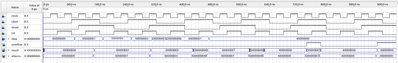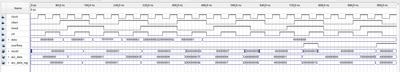FPGA Intellectual Property
PCI Express*, Networking and Connectivity, Memory Interfaces, DSP IP, and Video IP
Announcements
6162 Discussions

## Accumulator IP to VerilogNovice
788 Views

Hi support,

I am trying porting an old desine on C3 to C10.

Then I met a problem on the IP conversion.

The accumulator is not supported on C10.

I attached the IP file for your reference.

And I am wondering if I can the following code to replace it,

module acc_19i_25o (

clken,

clock,

data,

result);

input  clken;

input  clock;

input [18:0] data;

output [25:0] result;

wire [25:0] sub_wire0;

wire [25:0] result = sub_wire0[25:0];

reg [25:0] acc_data;

always@(posedge clock)

begin

if(clken) begin

acc_data <= acc_data + data;

end

end

assign sub_wire0 = acc_data ;

Thank you!

BRs,

Johnson

1 SolutionEmployee
542 Views

Hi @JLee25 ,

I found out there's bug on overflow triggering for signed custom code posted before. I had fixed the problem. I found this link http://www.pldworld.com/_altera/html/_sw/q2help/source/mega/mega_file_altaccumulate.htm and had followed the altaccumulate megafunction truth table which shown in red circle of image below.Here is the updated code (highlighted bold are changes):

module acc_19i_25o2 (

clken,

clock,

data,

cin,

result,

overflow

//result_prev_out,

//data_prev_out

);

input clken;

input clock;

input signed [30:0] data;

input cin;

output signed [30:0] result;

output overflow;

//output signed [30:0] result_prev_out;

//output signed [30:0] data_prev_out;

wire signed [30:0] sub_wire0;

wire signed [30:0] result = sub_wire0[30:0];

reg signed [30:0] acc_data;

reg signed [30:0] acc_data_reg;

reg signed [30:0] result_prev;

reg signed [30:0] data_prev;

reg overflow_reg;

always @(posedge clock) begin

if (clken) begin

if (result_prev != acc_data) begin // check if result has changed

result_prev <= acc_data; //previous result

end

if (data_prev != data ) begin // check if data has changed

data_prev <= data; //previous data

end

end

acc_data <= data;

acc_data_reg <= acc_data;

if ((data_prev<0&result_prev<0&acc_data>=0) | (data_prev>=0&result_prev>=0&acc_data<0)) begin

overflow_reg <= 1'b1;

end else begin

overflow_reg <= 1'b0;

end

end else if (clken) begin

acc_data <= acc_data + data + cin;

acc_data_reg <= acc_data;

if ((data_prev<0&result_prev<0&acc_data>=0) | (data_prev>=0&result_prev>=0&acc_data<0)) begin

overflow_reg <= 1'b1;

end else begin

overflow_reg <= 1'b0;

end

end else begin

acc_data <= acc_data;

acc_data_reg <= acc_data_reg;

overflow_reg <= overflow_reg;

end

end

assign sub_wire0 = acc_data_reg;

assign overflow = overflow_reg;

//assign result_prev_out = result_prev;

//assign data_prev_out = data_prev;

endmodule

Note:

a: data, data_prev

previous result: result_prev

result: acc_data

I had tested the custom code and altaccumulate megafunction (SIGNED, 1 cycle latency, Width 31) for 3 simulations. Simulation results and folder are attached below. The results are all exactly the same. The altaccumulate megafunction that I used don't have add_sub port. You may further verify from your end and let me know if there's any further concern.

Thanks,

Best Regards,

Sheng

12 RepliesEmployee
765 Views

Hi,

Yes. The altaccumulate Megafunction is not supported on C10. I think you probably can't replace the altaccumulate Megafunction with the code posted above because both of them having different simulation result check below.

altaccumulate Megafunction simulation (image):Code posted above simulation (image):Thanks,

Best Regards,

ShengNovice
754 Views

Hi Sheng,

Thanksfor getting back!

I change the design following the simulation result below,

module acc_19i_25o (

clken,

clock,

data,

result);

input  clken;

input  clock;

input [18:0] data;

output [25:0] result;

wire [25:0] sub_wire0;

wire [25:0] result = sub_wire0[25:0];

reg [25:0] acc_data;

always@(posedge clock)

begin

acc_data <= data;

else if(clken)

acc_data <= accdata + data;

else

acc_data <= accdata

end

assign sub_wire0 = acc_data ;

Thank you.

BRs,

JohnsonEmployee
742 Views

Hi Johnson,

With the updated code posted and minor syntax error modified below:

always@(posedge clock)

begin

acc_data <= data;

end else if(clken)

acc_data <= acc_data + data;

else

acc_data <= acc_data;

end

I'm getting the exactly same simulation result as altaccumulate Megafunction check image:Try with other input values also getting the same simulation for both updated code and altaccumulate Megafunction check image:Thanks,

Best Regards,

ShengNovice
720 Views

Hi Sheng,

Thank you very much.

By the way, I would like to address on the EXTRA_LATENCY in my original design file.

Do you think the simulation has this parameter included?

BRs,

JohnsonNovice
713 Views

Hi Sheng,

In the sample desing, I have another instance with new parameters like,

altaccumulate_component.extra_latency = 1,
altaccumulate_component.lpm_representation = "SIGNED",
altaccumulate_component.lpm_type = "altaccumulate",
altaccumulate_component.width_in = 31,
altaccumulate_component.width_out = 31;

I attached the file for your reference.

Any suggestions on how to replace it?

Thank you!

BRs,

JohnsonEmployee
692 Views

Hi Johnson,

Below is the updated verilog code for input/output width: 31, lpm_representation: UNSIGNED, extra_latency: 1 cycle

[Edited: below code is for UNSIGNED]

module acc_19i_25o2 (

clken,

clock,

data,

cin,

result,

overflow

);

input clken;

input clock;

input [30:0] data;

input cin;

output [30:0] result;

output reg overflow;

wire [30:0] sub_wire0;

wire [30:0] result = sub_wire0[30:0];

reg [30:0] acc_data;

reg [30:0] acc_data_reg;

reg overflow_reg;

always @(posedge clock) begin

acc_data <= data;

acc_data_reg <= acc_data;

overflow_reg <= 1'b0;

overflow <= overflow_reg;

end else if (clken) begin

{overflow_reg, acc_data} <= acc_data + data + cin;

overflow <= overflow_reg;

acc_data_reg <= acc_data;

end else begin

acc_data <= acc_data;

acc_data_reg <= acc_data_reg;

overflow_reg <= overflow_reg;

end

end

assign sub_wire0 = acc_data_reg;

endmodule

The code above being simulated without problem (same result with altaccumulate Megafunction):

altaccumulate Megafunction simulation (input/output width: 31, lpm_representation: UNSIGNED, extra_latency: 1 cycle):Custom verilog code simulation (input/output width: 31, lpm_representation: UNSIGNED, extra_latency: 1 cycle):1.Change if (sload) begin to if (sload && clken) begin (previous code needs this change as well)

2.Added cin, registered overflow, registered acc_data (extra latency)

3.Input/output width

4.UNSIGNED data representation

Thanks,

Best Regards,

ShengNovice
614 Views

Hi Sheng,

Thank you!

But would you check if this code has "extra_latency=1" ?

I don't see this behavior on the simulation result followed.

BRs,

JohnsonEmployee
658 Views

Hi Johnson,

For your reference, below is the verilog code for lpm_representation: SIGNED with input/output width: 31 and extra_latency: 1 cycle:

module acc_19i_25o2 (

clken,

clock,

data,

cin,

result,

overflow

);

input clken;

input clock;

input signed [30:0] data;

input cin;

output signed [30:0] result;

output overflow;

wire signed [30:0] sub_wire0;

wire signed [30:0] result = sub_wire0[30:0];

reg signed [30:0] acc_data;

reg signed [30:0] acc_data_reg;

reg overflow_reg;

always @(posedge clock) begin

acc_data <= data;

acc_data_reg <= acc_data;

if ((acc_data_reg>0&data>0&acc_data<0) | (acc_data_reg<0&data<0&acc_data>=0)) begin //use >= instead of >

overflow_reg <= 1'b1;

end else begin

overflow_reg <= 1'b0;

end

end else if (clken) begin

acc_data <= acc_data + data + cin;

if ((acc_data_reg>0&data>0&acc_data<0) | (acc_data_reg<0&data<0&acc_data>=0)) begin //use >= instead of >

overflow_reg <= 1'b1;

end else begin

overflow_reg <= 1'b0;

end

acc_data_reg <= acc_data;

end else begin

acc_data <= acc_data;

acc_data_reg <= acc_data_reg;

overflow_reg <= overflow_reg;

end

end

assign sub_wire0 = acc_data_reg;

assign overflow = overflow_reg;

endmodule

The simulation result of code above is same with altaccumulate Megafunction:

altaccumulate Megafunction simulation (input/output width: 31, lpm_representation: SIGNED, extra_latency: 1 cycle):Custom verilog code simulation (input/output width: 31, lpm_representation: SIGNED, extra_latency: 1 cycle):Thanks,

Best Regards,

ShengNovice
580 Views

Hi Sheng,

Thank you for the signed code..

But I am curious on the data<0 condition...BRs,

JohnsonEmployee
610 Views

Hi Johnson,

I had posted verilog code for input/output width: 31, lpm_representation: UNSIGNED, extra_latency: 1 cycle and input/output width: 31, lpm_representation: SIGNED, extra_latency: 1 cycle

I had compared the simulation result of custom verilog code with altaccumulate Megafunction having input/output width: 31, lpm_representation: UNSIGNED/SIGNED, extra_latency: 1 cycle. Both custom verilog code and altaccumulate Megafunction having exactly same simulation, I had attached the image in previous post.

Thanks,

Best Regards,

ShengEmployee
594 Views

Attached the design folder below for your reference (Using Quartus Standard v22.1).

waveform1.vwf for accum.v (altaccumulate Megafunction)

waveform.vwf for acc_19i_25o2_signed.v & acc_19i_25o2_unsigned.v (Both module name are acc_19i_25o2)

(**Note: In .vwf file, remember to change the path in Simulation -> Simulation Settings -> Timing Simulation Settings)

Thanks,

B.Regards,

ShengEmployee
543 Views

Hi @JLee25 ,

I found out there's bug on overflow triggering for signed custom code posted before. I had fixed the problem. I found this link http://www.pldworld.com/_altera/html/_sw/q2help/source/mega/mega_file_altaccumulate.htm and had followed the altaccumulate megafunction truth table which shown in red circle of image below.Here is the updated code (highlighted bold are changes):

module acc_19i_25o2 (

clken,

clock,

data,

cin,

result,

overflow

//result_prev_out,

//data_prev_out

);

input clken;

input clock;

input signed [30:0] data;

input cin;

output signed [30:0] result;

output overflow;

//output signed [30:0] result_prev_out;

//output signed [30:0] data_prev_out;

wire signed [30:0] sub_wire0;

wire signed [30:0] result = sub_wire0[30:0];

reg signed [30:0] acc_data;

reg signed [30:0] acc_data_reg;

reg signed [30:0] result_prev;

reg signed [30:0] data_prev;

reg overflow_reg;

always @(posedge clock) begin

if (clken) begin

if (result_prev != acc_data) begin // check if result has changed

result_prev <= acc_data; //previous result

end

if (data_prev != data ) begin // check if data has changed

data_prev <= data; //previous data

end

end

acc_data <= data;

acc_data_reg <= acc_data;

if ((data_prev<0&result_prev<0&acc_data>=0) | (data_prev>=0&result_prev>=0&acc_data<0)) begin

overflow_reg <= 1'b1;

end else begin

overflow_reg <= 1'b0;

end

end else if (clken) begin

acc_data <= acc_data + data + cin;

acc_data_reg <= acc_data;

if ((data_prev<0&result_prev<0&acc_data>=0) | (data_prev>=0&result_prev>=0&acc_data<0)) begin

overflow_reg <= 1'b1;

end else begin

overflow_reg <= 1'b0;

end

end else begin

acc_data <= acc_data;

acc_data_reg <= acc_data_reg;

overflow_reg <= overflow_reg;

end

end

assign sub_wire0 = acc_data_reg;

assign overflow = overflow_reg;

//assign result_prev_out = result_prev;

//assign data_prev_out = data_prev;

endmodule

Note:

a: data, data_prev

previous result: result_prev

result: acc_data

I had tested the custom code and altaccumulate megafunction (SIGNED, 1 cycle latency, Width 31) for 3 simulations. Simulation results and folder are attached below. The results are all exactly the same. The altaccumulate megafunction that I used don't have add_sub port. You may further verify from your end and let me know if there's any further concern.

Thanks,

Best Regards,

Sheng